why

• 累计撰写 197 篇文章
• 累计创建 11 个标签
• 累计收到 114 条评论

# 初看一脸懵逼，看懂直接跪下！2022-04-18 / 0 评论 / 0 点赞 / 517 阅读 / 5,353 字https://stackoverflow.com/questions/15182496/why-does-this-code-using-random-strings-print-hello-world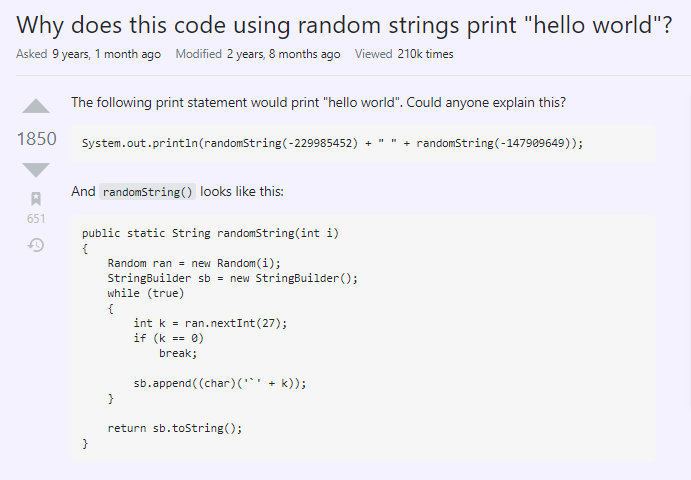public class MainTest {

public static void main(String[] args) {
System.out.println(randomString(-229985452) + " " + randomString(-147909649));
}

public static String randomString(int i) {
Random ran = new Random(i);
StringBuilder sb = new StringBuilder();
while (true) {
int k = ran.nextInt(27);
if (k == 0)
break;

sb.append((char) ('' + k));
}
return sb.toString();
}
}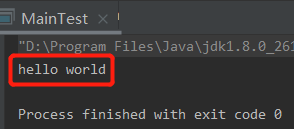## 高赞回答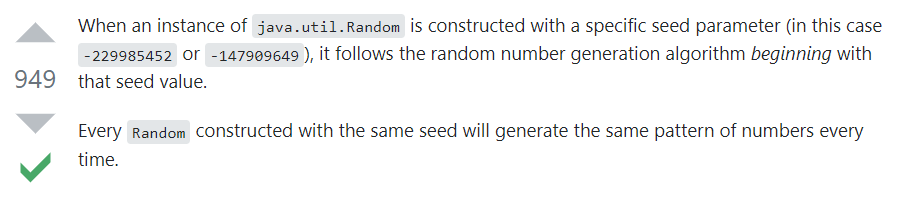public static void main(String[] args) {

randomString(-229985452);
System.out.println("------------");
randomString(-229985452);

}

private static void randomString(int i) {
Random ran = new Random(i);
System.out.println(ran.nextInt());
System.out.println(ran.nextInt());
System.out.println(ran.nextInt());
System.out.println(ran.nextInt());
System.out.println(ran.nextInt());

}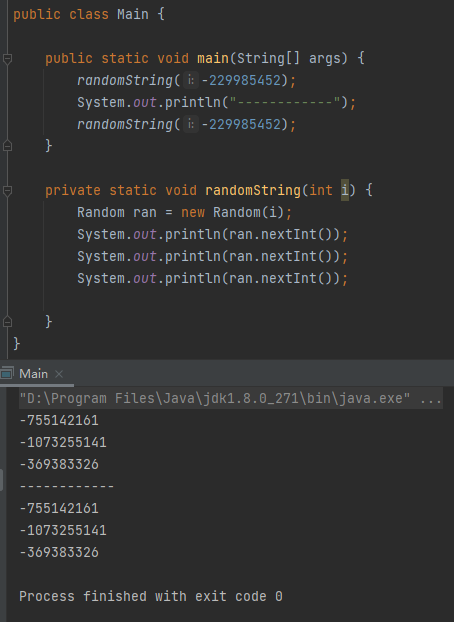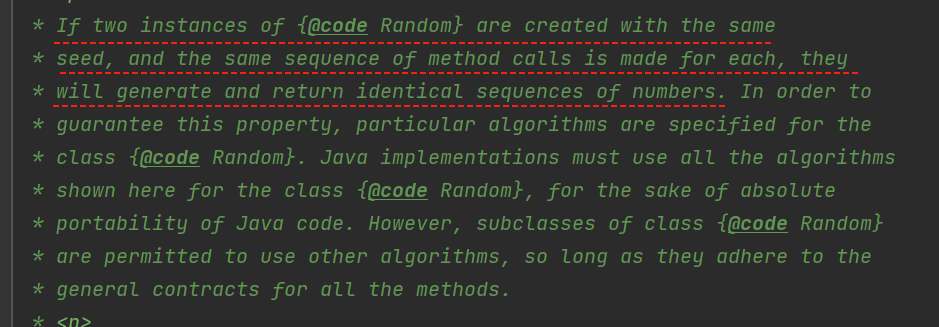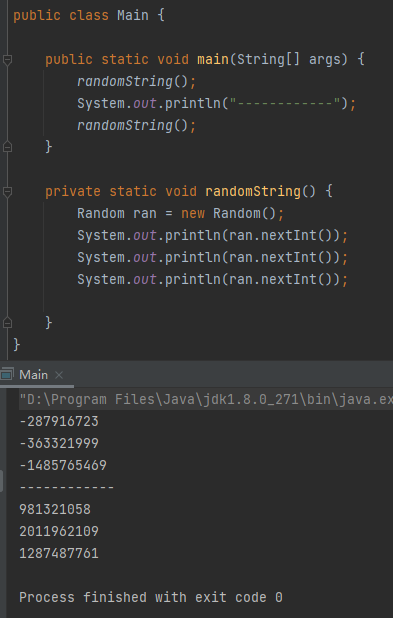## 再看看问题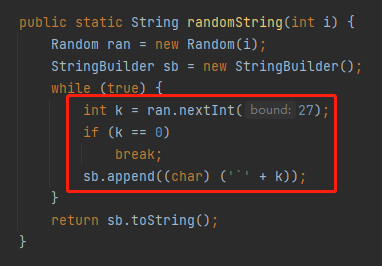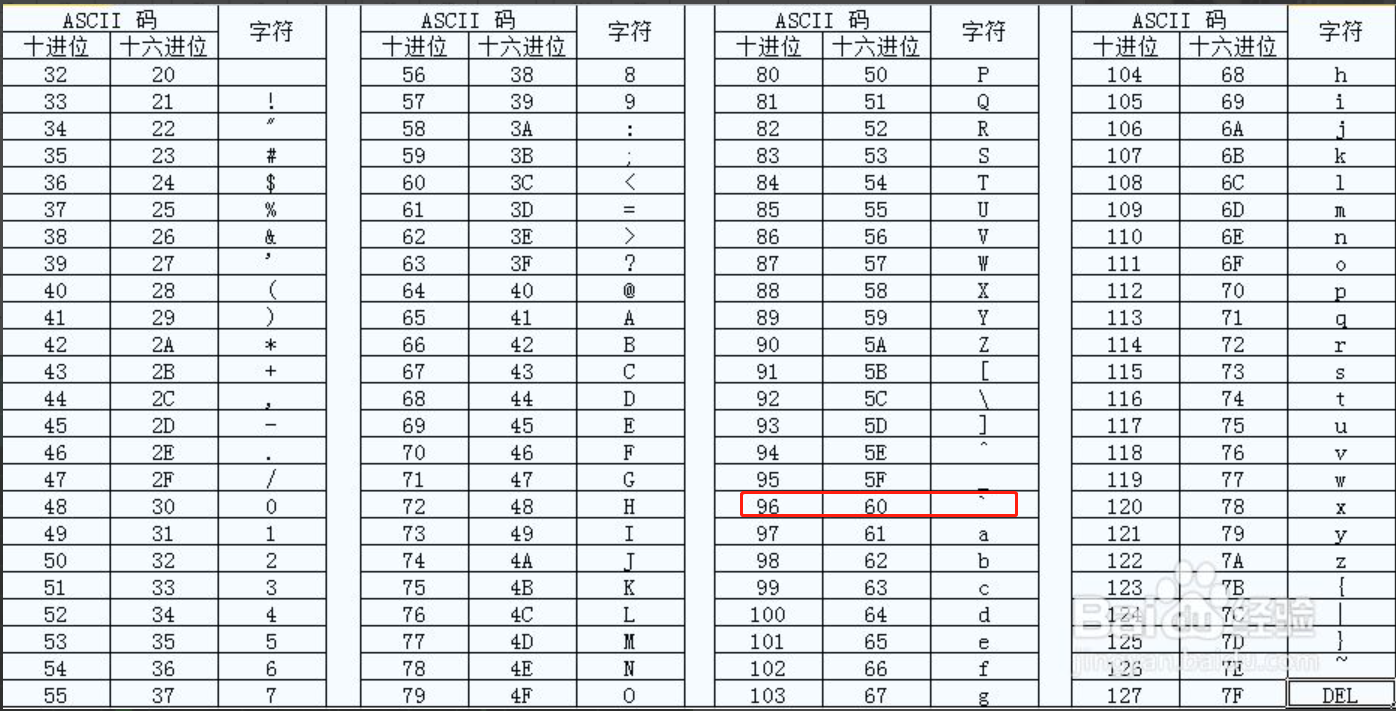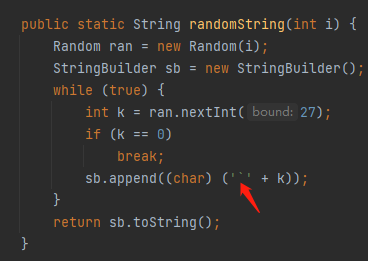'' + k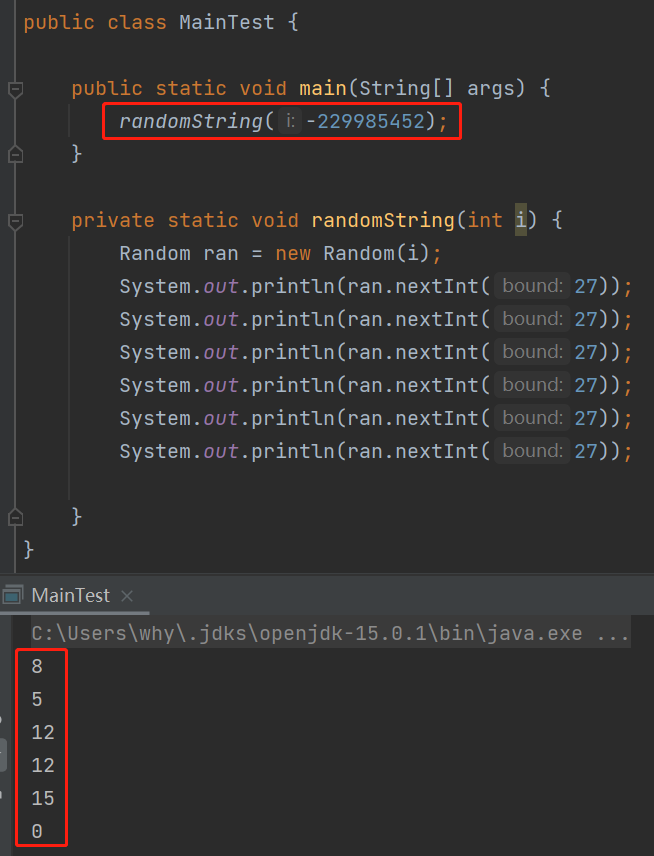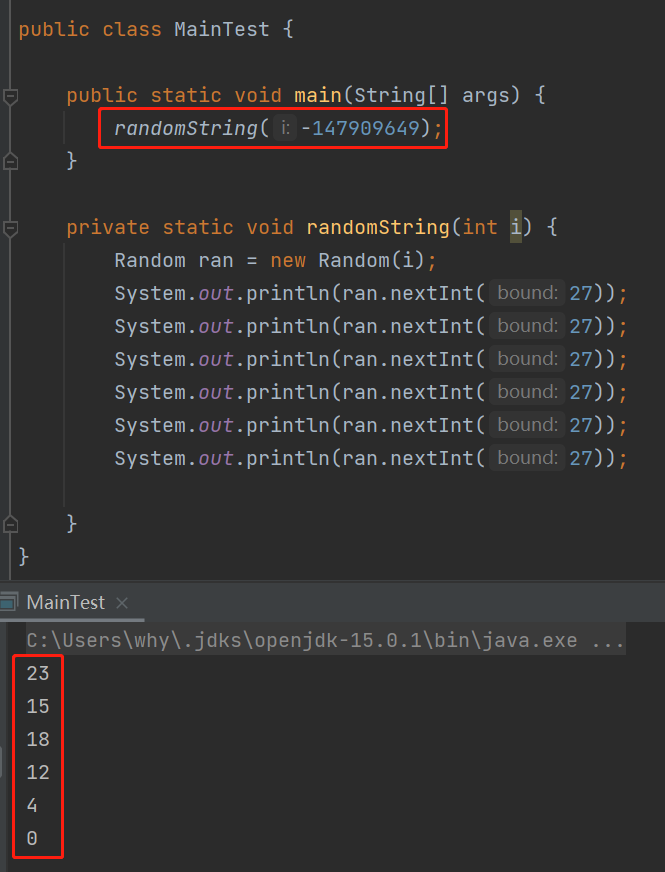8 + 96 = 104 --> h

5 + 96 = 101 --> e

12 + 96 = 108 --> l

12 + 96 = 108 --> l

15 + 96 = 111 --> o

23 + 96 = 119 --> w

15 + 96 = 111 --> o

18 + 96 = 114 --> r

12 + 96 = 108 --> l

4 + 96 = 100 --> d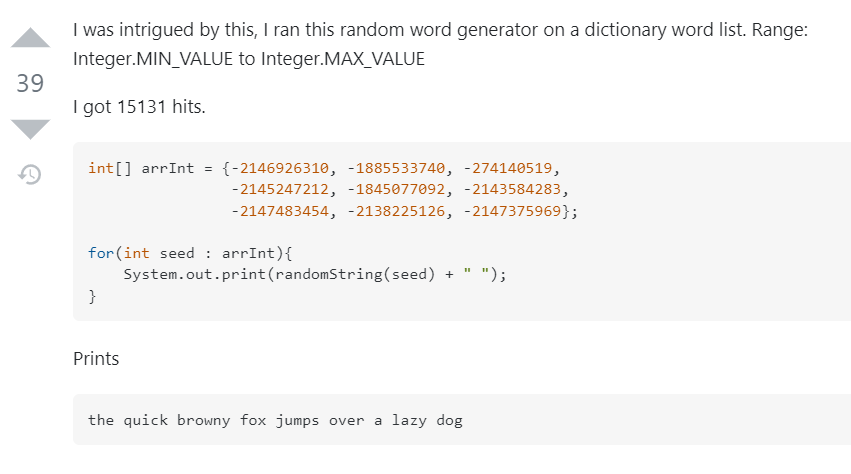public static long generateSeed(String goal, long start, long finish) {
char[] input = goal.toCharArray();
char[] pool = new char[input.length];
label:
for (long seed = start; seed < finish; seed++) {
Random random = new Random(seed);

for (int i = 0; i < input.length; i++)
pool[i] = (char) (random.nextInt(27) + '');

if (random.nextInt(27) == 0) {
for (int i = 0; i < input.length; i++) {
if (input[i] != pool[i])
continue label;
}
return seed;
}
}
throw new NoSuchElementException("Sorry :/");
}
`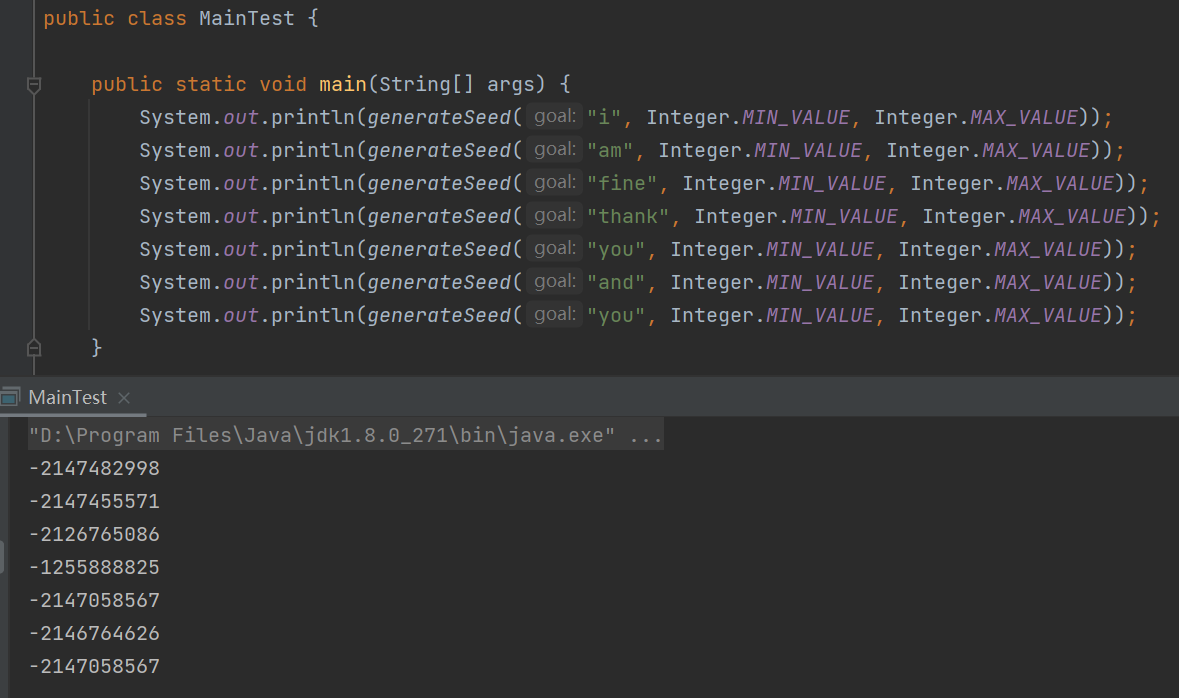## 源码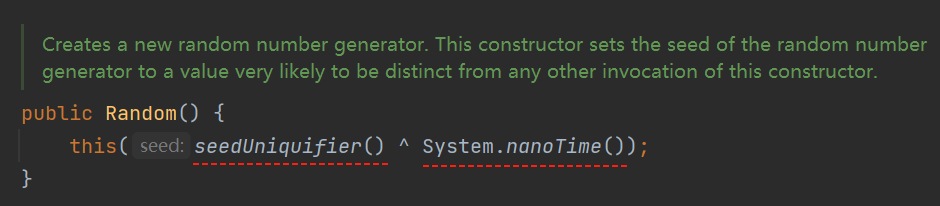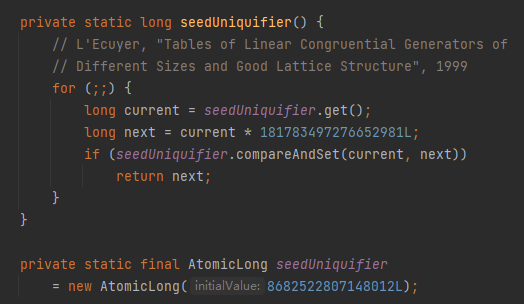181783497276652981L
8682522807148012L

https://stackoverflow.com/questions/18092160/whats-with-181783497276652981-and-8682522807148012-in-random-java-7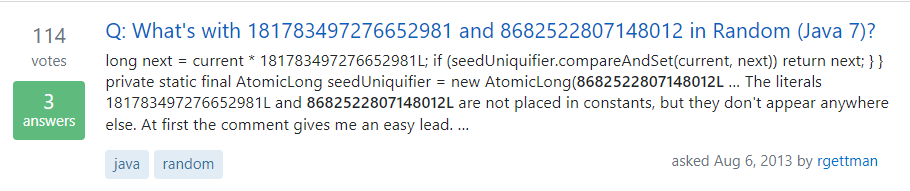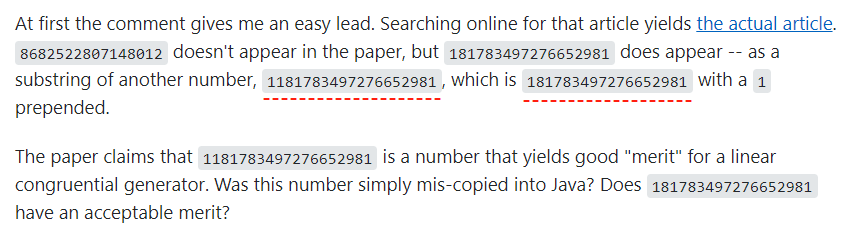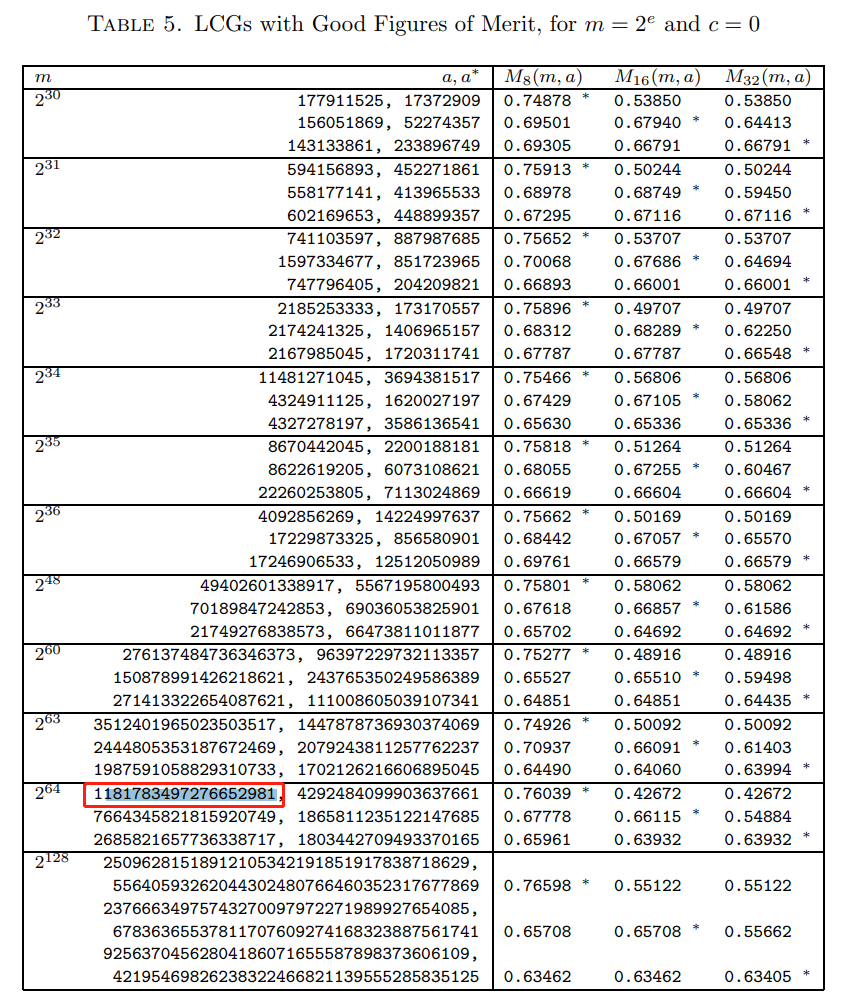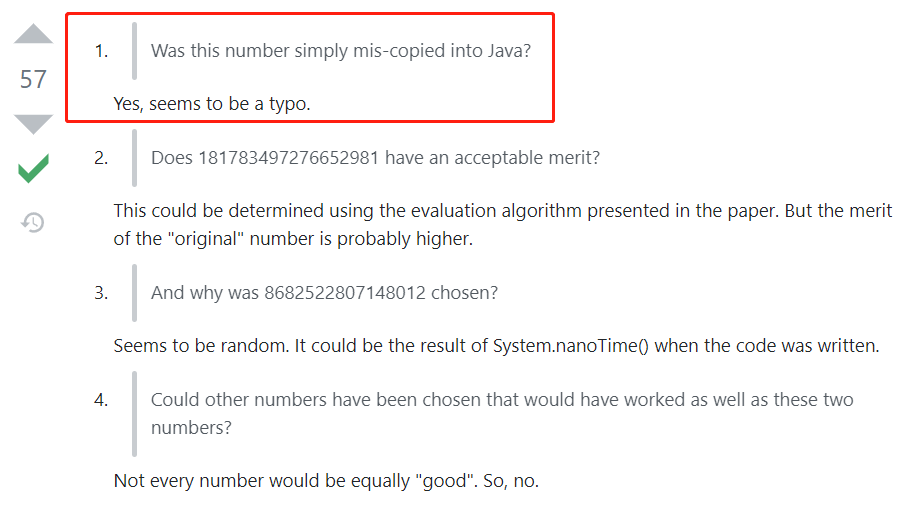“看起来确实像是拷错了。”https://bugs.openjdk.java.net/browse/JDK-8201634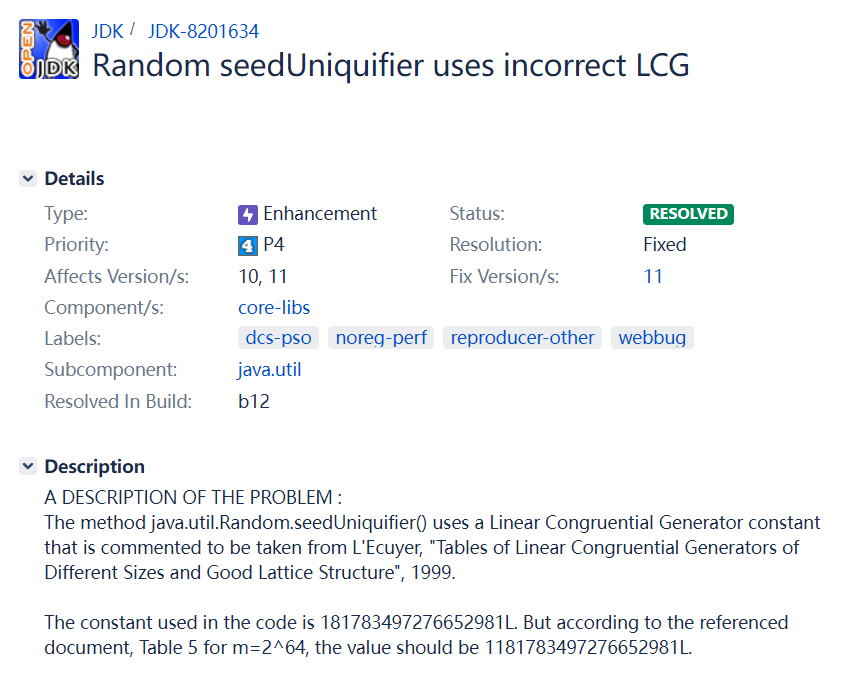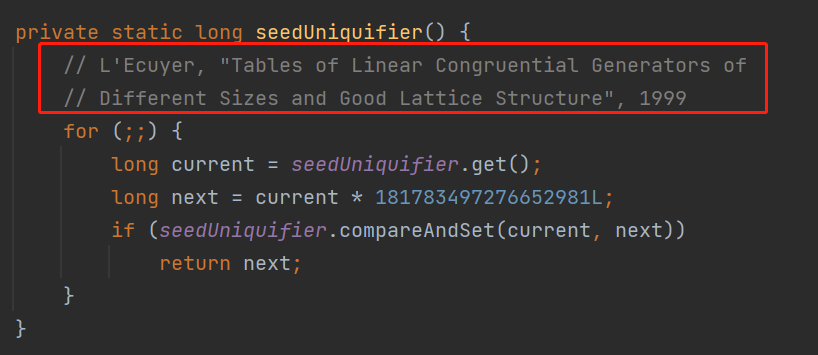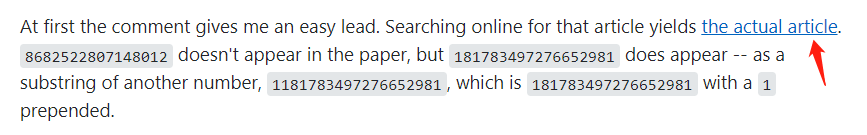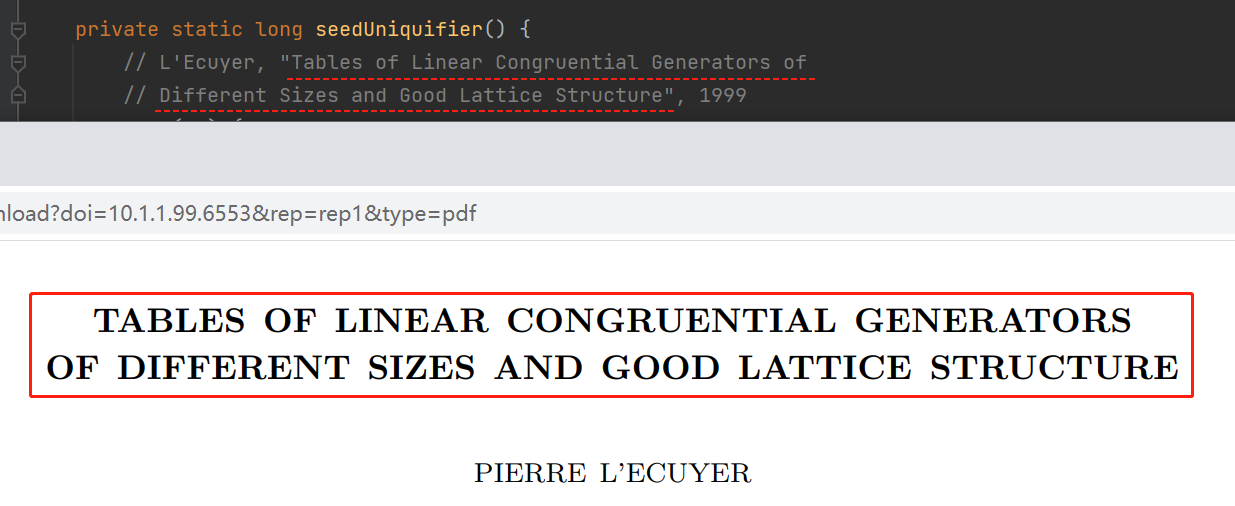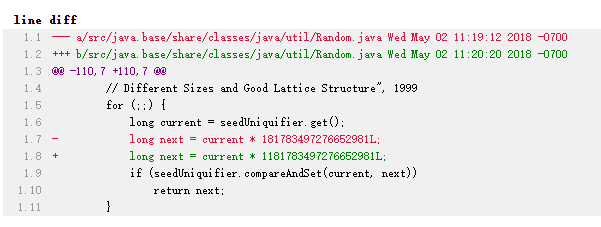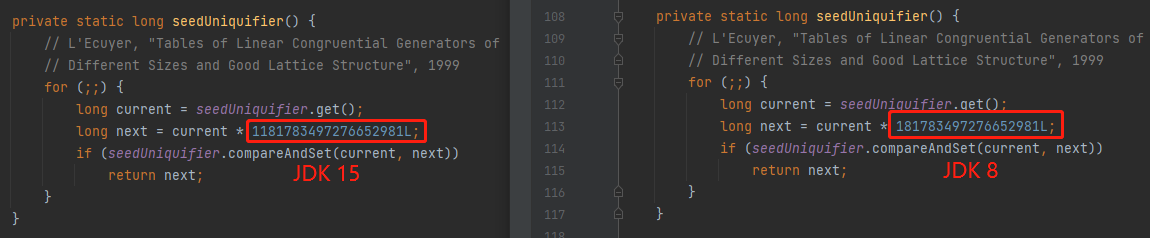## 荒腔走板0